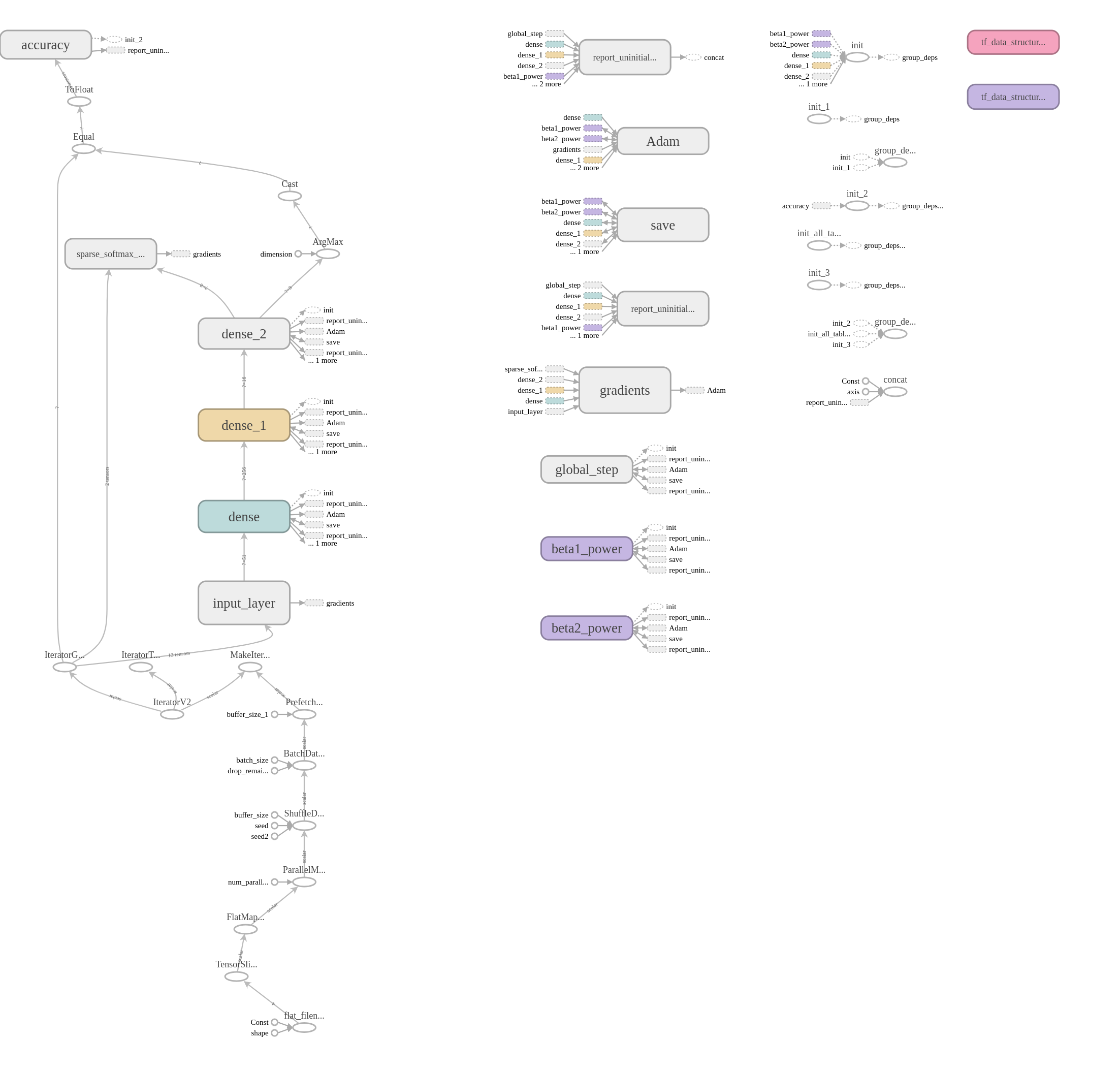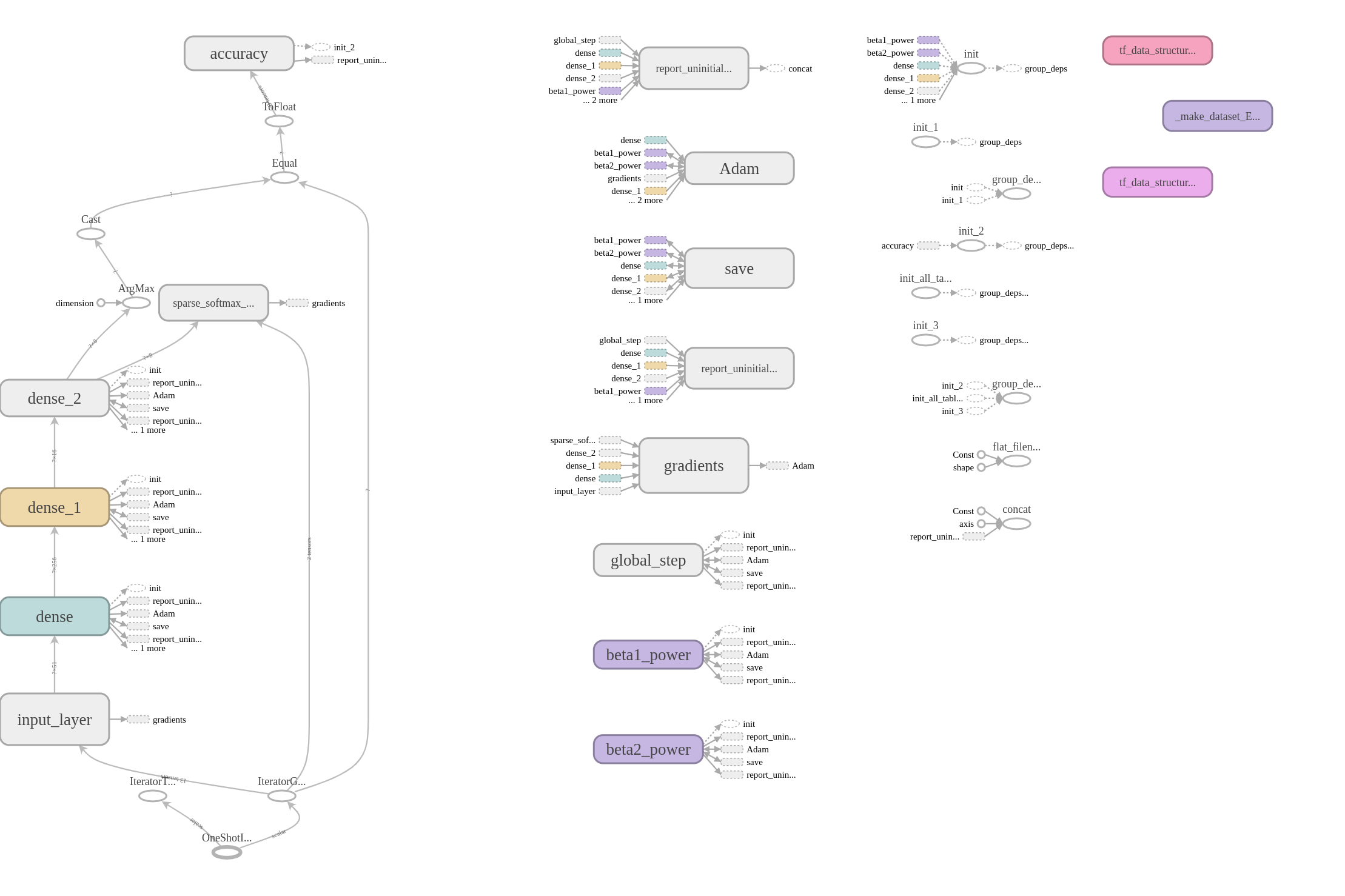07 Jan 2019

# Foreword

I refactored most of the utility functions from the previous tutorial code into a utility file.

## Changes

### 1) FeatureProto

1) removed CSV support - tfRecord all the way

2) The parser prototypes (tf.FixedLenFeature) are generated on initialization

3) Added an option to generate a One-Hot encoding while parsing the dataset

### 2) dataset_config

1) Added a return_dataset arg whereby instead of returning an iterator.get_next(), we return a configured tf.Dataset.

# Motivation

1) Show you how to use the generated tfRecord for training and inference

2) Give you an idea of how to use tf.estimators

# Story time

To recap, you’ve created one portion of the pipeline: you’ve verified that data is being transformed from .data format into a tfrecord. But now you’ve got to figure out how to USE the data before moving into productionizing all of it.

Given how the company is still moving away from Framework X, you’ve got a blank slate and no technical debt. Your good friend, Ian, recommended that you try a custom tf.estimators because it allows you to focus on building the model and doing your research and development, instead of focusing on “inference time” or “model serving” since all of those things are built in.

After all, you chose Tensorflow because it’s supposed to be the better option when scaling and serving, right? If you’re not going to use those features, and you’re going to write everything from scratch you should have used PyTorch.

# The plan

1) Give you a quick overview of how we’re loading the data (simple stuff since it’s not distributed)

2) Try a pre-made estimator. Cool, but not cool enough

3) Custom estimator

The code can be found here

def wrap_training_data(tf_record_train_list, feature_proto, num_cpus):
def input_fn_train(): # returns x, y (where y represents label's class index).
return dataset_config(filenames=tf_record_train_list, batch_size=64, mapper=feature_proto.unpack, num_cpus=num_cpus, return_dataset=True)
return input_fn_train

input_fn_train = wrap_training_data(filename_list, feature_proto, num_cpus)

def evaluate(estimator):
# Fit model.
fit = estimator.evaluate(input_fn=input_fn_eval)
print(fit)
estimator.train(input_fn=input_fn_train)
fit = estimator.evaluate(input_fn=input_fn_eval)
print(fit)


First, we wrap our input_fn_train data such that we can specify the config we want. The input_fn argument to the estimator.evaluate takes no arguments so we did it this way.

Notice how we used our feature_proto.unpack for the mapping function.

### 1.1) Note:

I chose to use return_dataset=True because it shows up explicitly in the graph. You can see this below:The default value is False and in that case you will get:but if you were to click on the one_shot_iterator, you’ll see some data

dataset_factory {"func":{"name":"_make_dataset_EdvWPMZGdR4"}}


where the factory is actually linked to the graph, but not in an explicit manner. I don’t know if there are any implications for it not being explicitly linked and it might be outside the scope of this tutorial. If you run your own experiments and come to a conclusion let me know and I’ll edit this!

There’s not much to it. I DID use a hacky method to suppress the training logs - it would print every 100 iterations so I did tf.logging.set_verbosity(tf.logging.ERROR) to hide it.

Results: okay

Results: great!

### 2.3) DNNLinearCombinedClassifier - without the Linear columns

results: surprising?

### Discussion

Looking at the implementation of DNNLinearCombinedClassifier it seems that , we see that it is almost similar to DNNClassifier (heck, it USES it), but somehow it does better. Good thing we did this study!

## 3) Custom Estimator

By abstracting well, you can effectively create a tf.estimator with little change to your current definitions.

### 3.1) Model Definition

def model_definition(features, feature_columns, labels):
"""

Params:
input_layer: tf.feature_column.input_layer

returns logits
"""

input_layer = tf.feature_column.input_layer(features, feature_columns)
layer_1 = tf.layers.Dense(256, activation=tf.nn.relu)(input_layer)
layer_2 = tf.layers.Dense(16, activation=tf.nn.relu)(layer_1)
logits = tf.layers.Dense(8)(layer_2)

# Define your prediction, loss, accuracy, and train_op
predictions = {'Class_ID': tf.argmax(input=logits, axis=1)}

loss = tf.losses.sparse_softmax_cross_entropy(labels=labels, logits=logits)
accuracy = tf.metrics.accuracy(labels, predictions['Class_ID'])

train_op = optimizer.minimize(loss, global_step=tf.train.get_global_step())
return predictions, loss, accuracy, train_op


Nothing much here to discuss - it looks more or less like any standard network except for the fact that

1) we wrap the predictions in a dictionary

2) we use a global_step. The global step lets us know how long this current model has been training for (even if we stopped it in between and such)

### 3.2) Boilerplate

def model_wrapper(feature_proto, abstract_model):
def custom_model(features, labels, mode):
if mode == tf.estimator.ModeKeys.PREDICT:
tf.logging.info("my_model_fn: PREDICT, {}".format(mode))
elif mode == tf.estimator.ModeKeys.EVAL:
tf.logging.info("my_model_fn: EVAL, {}".format(mode))
elif mode == tf.estimator.ModeKeys.TRAIN:
tf.logging.info("my_model_fn: TRAIN, {}".format(mode))

predictions, loss, accuracy, train_op = model_definition(
features, feature_proto.get_feature_columns(), labels
)

# Prediction
if mode == tf.estimator.ModeKeys.PREDICT:
return tf.estimator.EstimatorSpec(mode, predictions=predictions)

if mode == tf.estimator.ModeKeys.EVAL:
return tf.estimator.EstimatorSpec(
mode,
loss=loss,
eval_metric_ops={'custom_accuracy': accuracy}
)

# Track the accuracy while in training mode
tf.summary.scalar('my_accuracy', accuracy)
return tf.estimator.EstimatorSpec( mode, loss=loss, train_op=train_op)
return custom_model

classifier = tf.estimator.Estimator(
model_fn=model_wrapper(feature_proto, model_definition),
model_dir='save_dir/custom/'
)


Since the model_fn arg of the tf.estimator only takes in 3 arguments: features, labels, and mode, to pass in any additional features we wrap it up so that the variables exist in the scope.

## Done!

You’ve got the model working and it’s doing fine for now. Time to fine-tune!

### Next Steps

0) If you’re REALLY gung-ho you can dig into why DNNClassifier is beaten by DNNLinearCombinedClassifier (without a linear portion) and let me know.

1) Modify the custom model such that it uses the linear data separately and improve on the model.

If you figure out 0) or 1) do let me know because I’ve been scratching my head!

1) Try to improve on the accuracy! You can modify dataset_config to take in an epoch argument which can be used like dataset = dataset.repeat(epoch). If you don’t feed in an epoch, it just repeats indefinitely

2) Get ready for the next tutorial!